# AP Calculus AB Practice Test 44

### Test Information9 questions27 minutes

Calculator Allowed

1.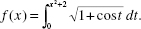Then f (x) =

2.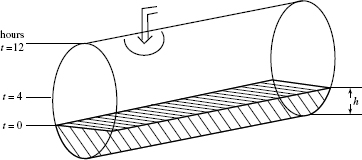A cylindrical tank, shown in the figure above, is partially full of water at timet = 0, when more water begins flowing in at a constant rate. The tank becomes halffull when t = 4, and is completely full when t = 12. Let h represent the height of the water at time t. During which interval is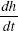increasing?

3. A particle moves on a line according to the law s = f (t) so that its velocity v = ks, where k is a nonzero constant. Its acceleration is

4. A cup of coffee placed on a table cools at a rate of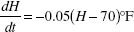per minute, where H represents the temperature of the coffee and t is time in minutes. If the coffee was at 120°F initially, what will its temperaturebe 10 minutes later?

5. An investment of \$4000 grows at the rate of 320e0.08t dollars per year after t years. Its value after 10 years is approximately

6. If f (x) = (1 + ex) then the domain of f -1(x) is

7. The graph below shows the velocity of an object during the interval 0 ≤ t ≤ 9.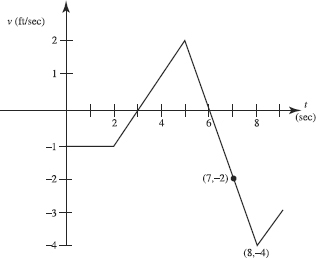The object attains its greatest speed at t =

8. The graph below shows the velocity of an object during the interval 0 ≤ t ≤ 9.The object was at the origin at t = 3. It returned to the origin

9. When the region bounded by the y-axis, y = ex, and y = 2 is rotated around the y-axis it forms a solid with volume